# If a hydrogen atom is initially in its n = 3 state, which of the following...

If a hydrogen atom is initially in its n = 3 state, which of the following frequencies can it emit a photon at? a) 0.57 x 10^14 Hz b) 1.57 x 10^14 Hz c) 2.57 x 10^14 Hz d) 3.57 x 10^14 Hz e) 4.57 x 10^14 Hz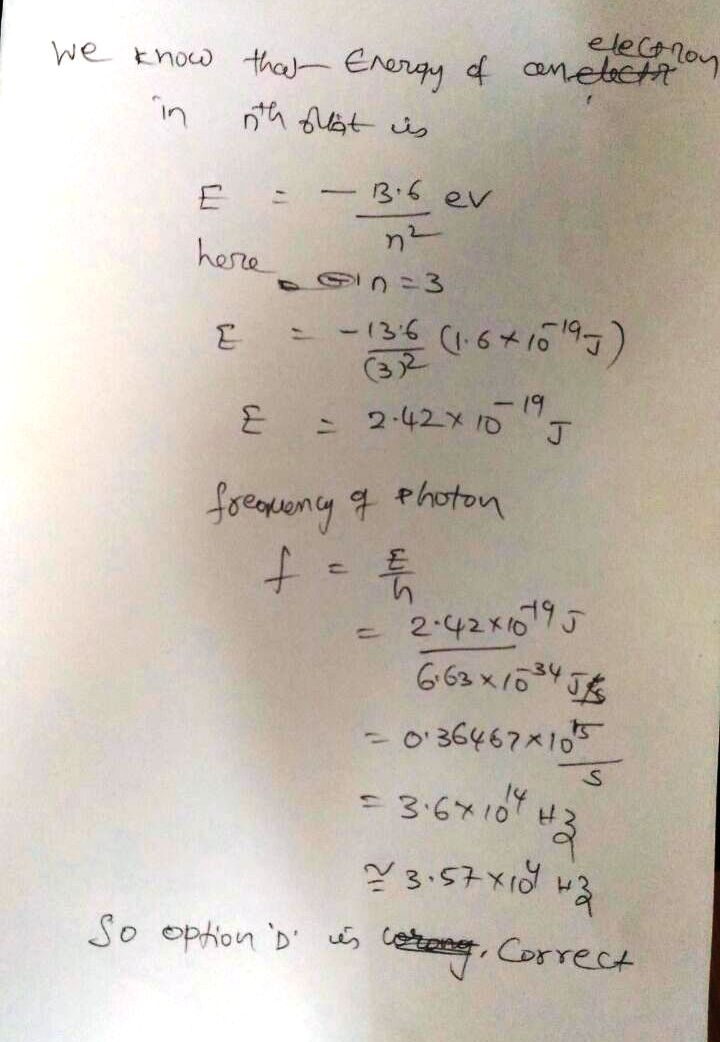#### Earn Coin

Coins can be redeemed for fabulous gifts.

Similar Homework Help Questions
• ### hydrogen atom n value

Determine the final value of n in a hydrogen atom, if the electron starts at n=2 and the atom absorbs a photon of light with a frequency of 4.57 x 10^14 Hz

• ### A hydrogen atom initially in its ground state i.e., n= 1 level, absorbs a photon and...

A hydrogen atom initially in its ground state i.e., n= 1 level, absorbs a photon and ends up in n= 3 level. What must have been the frequency of the incident photon? (b) Now the electron makes spontaneous emission and comes back to the ground state. What are the possible frequencies of the photons emitted during this process?

• ### A hydrogen atom initially in its ground state absorbs a photon and ends up in the...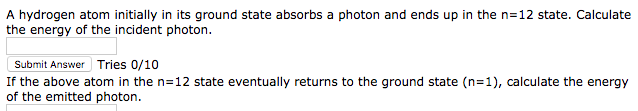A hydrogen atom initially in its ground state absorbs a photon and ends up in the n=12 state. Calculate the energy of the incident photon Submit Answer Tries 0/10 If the above atom in the n=12 state eventually returns to the ground state (n=1), calculate the energy of the emitted photon.

• ### Determine the end (final) value of n in a hydrogen atom transition, if the electron starts in n...

Determine the end (final) value of n in a hydrogen atom transition, if the electron starts in n = 2 and the atom absorbs a photon of light with a frequency of 4.57 ×10 14 Hz.

• ### A hydrogen atom initially in the ground level absorbs a photon, which excites it to the...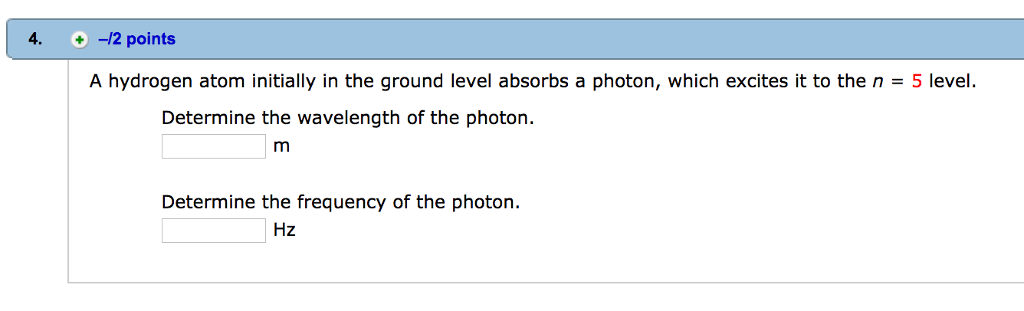A hydrogen atom initially in the ground level absorbs a photon, which excites it to the n = 5 level. Determine the wavelength of the photon. m Determine the frequency of the photon. Hz

• ### A hydrogen atom initially in the ground level absorbs a photon, which excites it to the...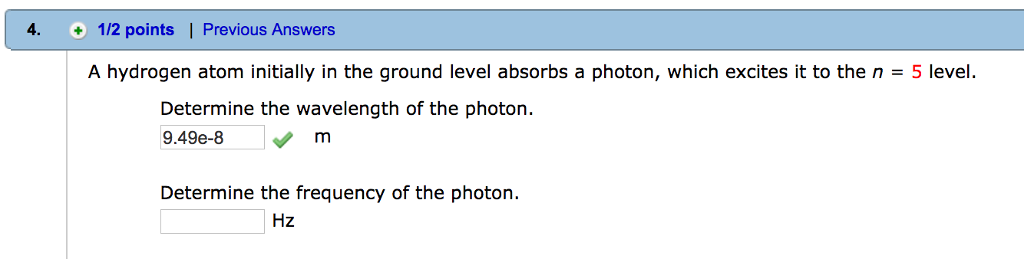A hydrogen atom initially in the ground level absorbs a photon, which excites it to the n = 5 level. Determine the wavelength of the photon. 9.49e-8 m Determine the frequency of the photon. Hz

• ### A hydrogen atom initially in the n=11 quantum state emits a photon having a wavelength of...

A hydrogen atom initially in the n=11 quantum state emits a photon having a wavelength of 0.886044E-6 m. What is the quantum number of the final state of the atom?

• ### Part A A hydrogen atom makes a transition from the n = 5 state to a...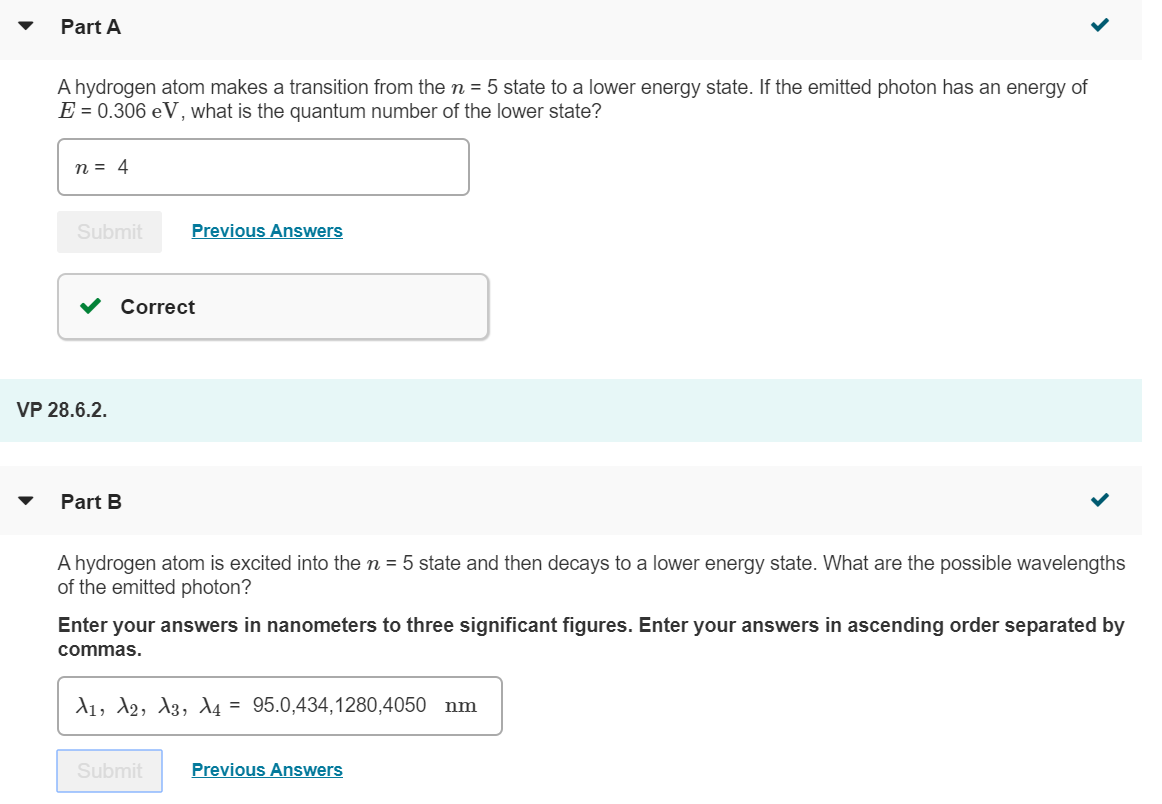Part A A hydrogen atom makes a transition from the n = 5 state to a lower energy state. If the emitted photon has an energy of E = 0.306 eV, what is the quantum number of the lower state? n = 4 Submit Previous Answers Correct VP 28.6.2. Part B A hydrogen atom is excited into the n = 5 state and then decays to a lower energy state. What are the possible wavelengths of the emitted photon? Enter...

• ### A hydrogen atom is excited from its ground state to the n = 3 state. The...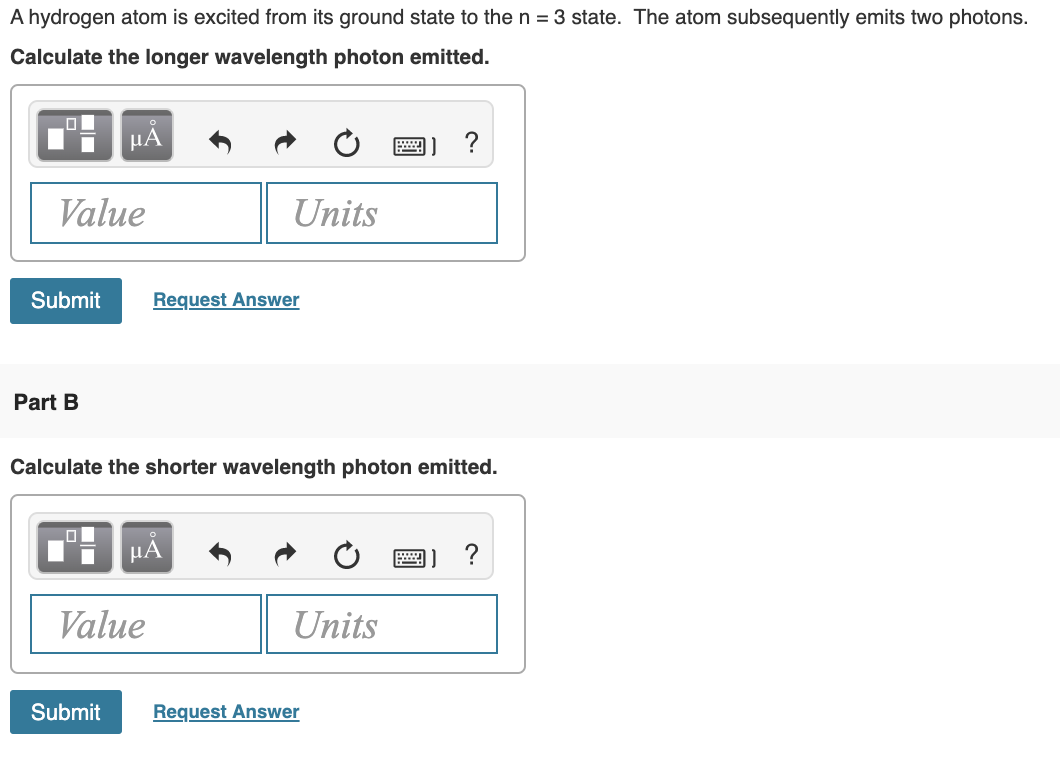A hydrogen atom is excited from its ground state to the n = 3 state. The atom subsequently emits two photons. Calculate the longer wavelength photon emitted. Value Units Submit Request Answer Part B Calculate the shorter wavelength photon emitted. MÅ O 2 ? Value Units Submit Request Answer

• ### 15. Which of the following transitions in a hydrogen atom would emit the highest energy photon?...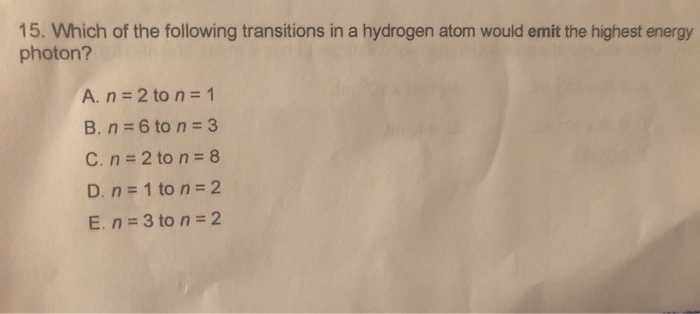15. Which of the following transitions in a hydrogen atom would emit the highest energy photon? A. n = 2 to n = 1 B. n = 6 to n = 3 C. n = 2 to n = 8 D. n = 1 to n = 2 E n = 3 to n = 2